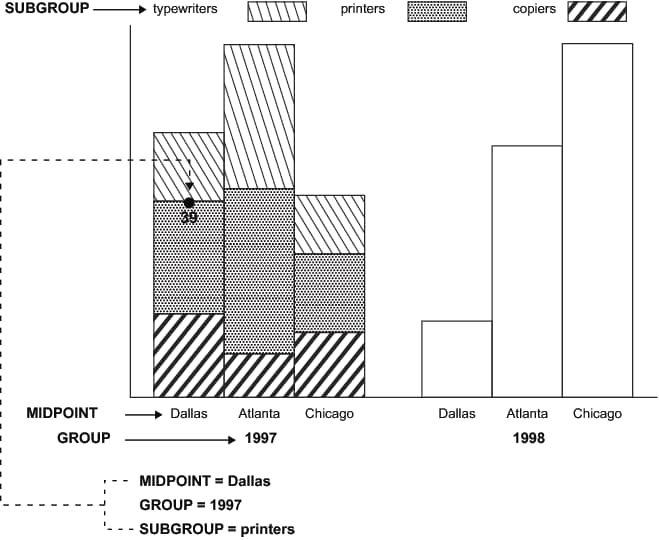# GROUP Variable

Positions graphics elements on the bars of a vertical or horizontal bar chart drawn using the GROUP= option in the GCHART procedure.
 Type: Numeric or character; must match the type of the GROUP= variable used in the GCHART procedure. Length: Should match the length of GROUP= variable in the GCHART procedure. Default: none Restriction: Used only with vertical or horizontal bar charts produced by the GCHART procedure.

## Syntax

GROUP=group-value;

### Variable Values

group-value
references value(s) of the variable that is identified by the GROUP= option in the GCHART procedure either as a variable name or as an explicit data value. Group-value can be one of the following:
group-variable
the name of a group variable.
group-data-value
a specific numeric data value.
'group-data-value'
a specific character data value.
To annotate all the bars in a horizontal or vertical bar chart, specify a variable name. To annotate a bar chart for a specific value of the GROUP variable, specify a specific value.

## Details

### Using the GROUP Variable

Using the GROUP variable is similar to using the X and Y variables with data system coordinates to position graphics elements in a vertical or horizontal bar chart.
shows how the GROUP variable works with the SUBGROUP and MIDPOINT variables to label the bars of a vertical bar chart.
Using the GROUP Variable to Position a Label in a Bar ChartThe label showing the number of units that were sold in Dallas in the year 1997 is positioned by the values that are assigned to these Annotate variables:
• GROUP=YEAR (where YEAR is a variable in the GCHART data set)
• MIDPOINT=CITY (where CITY is a variable in the GCHART data set)
• SUBGROUP=ITEM (where ITEM is a variable in the GCHART data set).

### Functions

You can use the GROUP variable only with the data coordinate systems 1, 2, 7, and 8, and with these functions:
 BAR MOVE POINT DRAW PIE SYMBOL LABEL PIECNTR